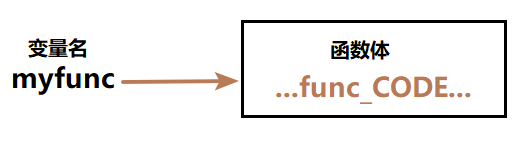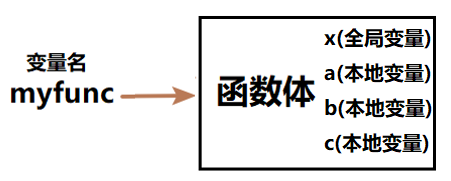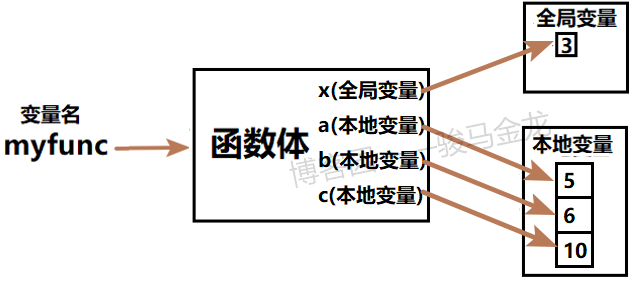# Python函数基础

## 函数声明、调用、返回基础

Python中使用def关键字来声明函数，声明函数的格式为：

def func_name(args):
...body...
[return ...]


1. 函数名后面必须加冒号
2. 如果函数体和def不在同一行，则必须缩进
3. return指定函数返回值，用来结束函数
• 但return语句是可有可无的，如果不给return，则等价于加上了return None，即函数默认返回None结构

def myfunc(x,y,z): print(x+y+z)


myfunc(1,2,3)


def func(x):
return x+5


print(func(3))    # 输出返回值

a=func(4)         # 赋值给变量
print(a)

print(func(5)+3)  # 数值操作


return语句是可选的，如果函数中不指定return语句，则默认返回None，即类似于return None

## 关于函数参数

def myfunc(x,y,z):
print(x,y,z)

myfunc(1,2,3)


def首先声明好函数，然后到了myfunc(1,2,3)时，表示调用函数(执行函数)，调用函数时会将给定的值1,2,3传递给函数的参数x,y,z，其实就是变量赋值x=1,y=2,z=3，然后使用print输出它们。

myfunc(1,2,3)
myfunc("abc",2,"def")
myfunc([1,2,3],4,5)


python函数的参数相比其它语言要复杂一些，意味着要灵活很多，短短一个小节的篇幅完全没法解释清楚，关于参数细节，详细内容见后面的文章

## 函数声明、调用的过程详述

def用来声明一个函数，python的函数包括函数名称、参数、函数体、函数体中涉及到的变量、返回值。

def myfunc(x,y,z):
...CODE...def myfunc(x):
return x+5

print(myfunc)


<function myfunc at 0x032EA6F0>


### 函数变量的细节

python是解释性语言，读一行解释一行，解释一行忘记一行。而函数是一种代码块，代码块是一个解释单元，是一个整体。在代码块范围内不会忘记读取过的行，也不会读一行就立即解释一行，而是读取完所有代码块内的行，然后统筹安排地进行解释。关于这一点，在后面的文章代码块详述中有非常详细的解释，建议一读。

x=3
def myfunc(a,b):
c=10
print(x,a,b,c)

myfunc(5,6)


• 全局变量x
• 本地变量a、b、c，其中本地变量a和b是函数的参数• 查找全局变量变量x，它在全局作用域内已经赋值过了，所以只需找到这个全局变量即可
• 查找本地变量a、b、c，它们是属于函数myfunc的本地变量，而a和b是参数变量，所以最先对它们进行赋值a=5,b=6，然后赋值普通的本地变量c=10x=3
def myfunc(a,b):
c=10
print(x,a,b,c)

x=33
myfunc(5,6)


## 匿名函数lambda

lambda声明匿名函数的方式很简单，lambda关键字后面跟上参数列表，然后一个冒号，冒号后跟一个表达式。

lambda argl, arg2,... argN :expression statement


lambda表达式返回一个匿名函数，这个匿名函数可以赋值给一个变量

# 声明匿名函数，并赋值给变量f
f = lambda x,y,z: x+y+z

print(f)


<function <lambda> at 0x027EA6F0>


# 调用匿名函数
print(f(2,3,4))  # 输出9


L=[ lambda x: x * 2,
lambda x: x * 3,
lambda x: x * 4 ]

print(L(2))
print(L(2))
print(L(2))


def f1(x): return x * 2
def f2(x): return x * 3
def f3(x): return x * 4

L=[f1,f2,f3]

print(L(2))
print(L(2))
print(L(2))


key='four'
print(
{
'two':(lambda x: x * 2),
'three':(lambda x: x * 3),
'four':(lambda x: x * 4)
}[key](2)
)


## 函数嵌套

def f(x):
y=10
def g(z):
return x+y+z
return g


# 将嵌套的函数赋值给变量myfunc
# 这时myfunc()和g()是等价的
myfunc = f(3)
print( myfunc(5) )

# 直接调用g()
print( f(3)(5) )


def f(x):
y=10
return lambda z: x+y+z


### 嵌套在循环内部的函数

def f():
L=[]
for i in range(5):
L.append( lambda : i )
return L


List = f()
print(List())
print(List())
print(List())
print(List())
print(List())


4
4
4
4
4


### 嵌套函数的作用域

x=3
def f():
x=4
g()
print("f:",x)

def g():
print("g:",x)

f()


g: 3
f: 4


x=3
def f():
x=4
g()
print("f:",x)

def g():
print("g:",x)

x=6
f()


g: 6
f: 4


x=3
def f():
x=4
def g():
print("g:",x)
print("f:",x)
x=5
return g

f()()


f: 4
g: 5


Linux运维交流群：921383787

posted @ 2018-11-09 00:47  骏马金龙  阅读(4228)  评论(0编辑  收藏  举报